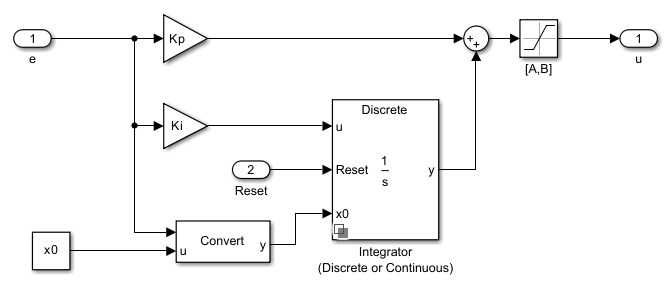# Discrete PI Controller with Integral Anti-Windup

Discrete-time PI control with integral anti-windup

•Libraries:
Simscape / Electrical / Control / General Control

## Description

The Discrete PI Controller with Integral Anti-Windup block implements discrete PI control with internal anti-windup. The figure shows the equivalent circuit for the controller with internal anti-windup.### Equations

The block calculates the control signal using the backward Euler discretization method:

`$u\left(k\right)=\text{sat}\left({K}_{p}\text{e}\left(\text{k}\right)+\text{sat}\left({K}_{i}\frac{{T}_{s}z}{z-1}\text{e}\left(\text{k}\right),\text{A},\text{B}\right),A,B\right),$`

where:

• u is the control signal.

• Kp is the proportional gain coefficient.

• e is the error signal.

• Ki is the integral gain coefficient.

• Ts is the sampling period.

• A is the lower limit for saturation.

• B is the upper limit for saturation.

## Ports

### Input

expand all

Error signal, e(k), obtained as the difference between the reference, r(k), and measurement y(k) signals.

Data Types: `single` | `double`

External reset (rising edge) signal for the integrator.

Data Types: `single` | `double`

### Output

expand all

Control signal, u(k).

Data Types: `single` | `double`

## Parameters

expand all

Proportional gain, Kp, of the PI controller.

Integral gain, Ki, of the PI controller.

Upper limit, B, of the output for the PI controller.

Lower limit, A, of the output for the PI controller.

Value of the integrator at simulation start time.

Time between consecutive block executions. During execution, the block produces outputs and, if appropriate, updates its internal state. For more information, see What Is Sample Time? and Specify Sample Time.

For inherited discrete-time operation, set this parameter to `-1`. For discrete-time operation, set this parameter to a positive integer. For continuous-time operation, set this parameter to `0`.

If this block is in a masked subsystem or a variant subsystem that supports switching between continuous operation and discrete operation, promote this parameter to ensure correct switching between the continuous and discrete implementations of the block. For more information, see Promote Block Parameters on a Mask.

 IEEE Recommended Practice for Excitation System Models for Power System Stability Studies. IEEE Std 421.5/D39. Piscataway, NJ: IEEE-SA, 2015.